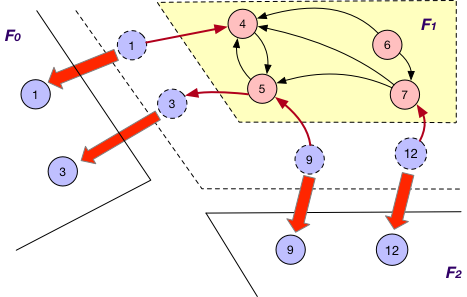GraphScope Analytical Engine

The analytical engine in GraphScope derives from GRAPE, a graph processing system proposed on SIGMOD-2017. GRAPE differs from prior systems in its ability to parallelize sequential graph algorithms as a whole. In GRAPE, sequential algorithms can be easily “plugged into” with only minor changes and get parallelized to handle large graphs efficiently. In addition to the ease of programming, GRAPE is designed to be highly efficient and flexible, to cope the scale, variety and complexity from real-life graph applications.

Built-in Algorithms

GraphScope analytical engine provides many common used algorithms, including connectivity and path analysis, community detection, centrality computations.

Built-in algorithms can be easily invoked over loaded graphs. For example,

import graphscope

graphscope.set_option(show_log=True)

# many algorithms only support evaluate on simple graph
# hence we need to generate one by selecting a kind of vertices and edges.
simple_g = g.project(vertices={"host": ["id"]}, edges={"connect": ["dist"]})

# run builtin applications
result_lpa = graphscope.lpa(simple_g, max_round=20)
result_sssp = graphscope.sssp(simple_g, src=20)

A full-list of builtin algorithms is shown as below. Whether an algorithm supports property graph or not is described in its docstring.

The list is continuously growing.

Result Processing

When finish a computation, the results are wrapped as Context and preserved on the distributed machines.

Users may want to fetch the results to the client, or write to cloud storage or distributed file systems.

There is a list of supported method to retrieve the results.

# fetch to data structures
result_lpa.to_numpy("r")
result_lpa.to_dataframe({"node": "v.id", "result": "r"})

# or write to hdfs or oss, or local (local means the path is relative to the pods)
result_lpa.output("hdfs://output", {"node": "v.id", "result": "r"})
result_lpa.output("oss://id:key@endpoint/bucket/object", {"node": "v.id", "result": "r"})
result_lpa.output("file:///tmp/path", {"node": "v.id", "result": "r"})

# or write to client local
result_lpa.output_to_client("/tmp/lpa_result.txt", {"node": "v.id", "result": "r"})

# or seal to vineyard
result_lpa.to_vineyard_dataframe({"node": "v.id", "result": "r"})
result_lpa.to_vineyard_tensor("r")

In addition, as shown in the Getting Started, computation results can add back to the graph as a new property (column) of the vertices(edges).

# add the results as new columns to the citation graph, the column name is 'lpa_result'

Users may assign a Selector to define which parts of the results to write. A selector specifies which part of the result to preserve. Similarly, the graph data can also be a part of the result, e.g., the vertex id. We reserve three keywords for selectors. r represents the result, v and e for vertices and edges, respectively. Here are some examples for selectors on result processing.

# get the results on the vertex
result_lpa.to_numpy('r')

# to dataframe,
# using the `id` of vertices (`v.id`) as a column named df_v
# using the `data` of v (`v.data`) as a column named df_vd
# and using the result (`r`) as a column named df_result
result_lpa.to_dataframe({'df_v': 'v.id', 'df_vd': 'v.data', 'df_result': 'r'})

# for results on property graph
# using `:` as a label selector for v and e
# using the id for vertices labeled with label0 (`v:label0.id`) as column `id`
# using the property0 written on vertices with label0 as column `result`
result.output(fd='hdfs:///gs_data/output', \
selector={'id': 'v:label0.id', 'result': 'r:label0.property0'})

See more details in Context and Selector.

Writing Your Own Algorithms in PIE

Users may write their own algorithms if the built-in algorithms do not meet their needs. graphscope enables users to write algorithms in the PIE programming model in a pure Python mode.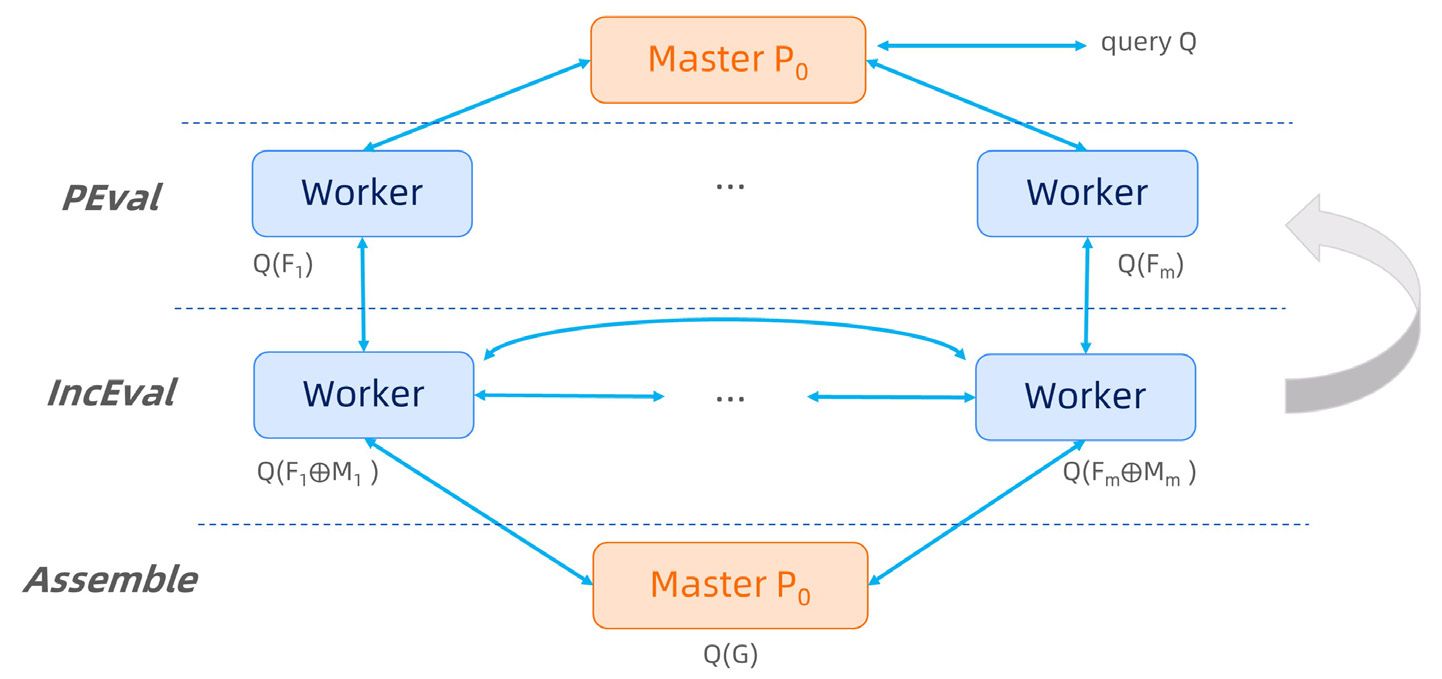To implement this, a user just need to fulfill this class.

from graphscope.analytical.udf.decorators import pie
from graphscope.framework.app import AppAssets

@pie(vd_type="double", md_type="double")
class YourAlgorithm(AppAssets):
@staticmethod
def Init(frag, context):
pass

@staticmethod
def PEval(frag, context):
pass

@staticmethod
def IncEval(frag, context):
pass

As shown in the code, users need to implement a class decorated with @pie and provides three sequential graph functions. In the class, the Init is a function to set the initial status. PEval is a sequential method for partial evaluation, and IncEval is a sequential function for incremental evaluation over the partitioned fragment. The full API of fragment can be found in Cython SDK API.

Let’s take SSSP as example, a user defined SSSP in PIE model may be like this.

from graphscope.analytical.udf.decorators import pie
from graphscope.framework.app import AppAssets

@pie(vd_type="double", md_type="double")
class SSSP_PIE(AppAssets):
@staticmethod
def Init(frag, context):
v_label_num = frag.vertex_label_num()
for v_label_id in range(v_label_num):
nodes = frag.nodes(v_label_id)
context.init_value(
nodes, v_label_id, 1000000000.0, PIEAggregateType.kMinAggregate
)
context.register_sync_buffer(v_label_id, MessageStrategy.kSyncOnOuterVertex)

@staticmethod
def PEval(frag, context):
src = int(context.get_config(b"src"))
graphscope.declare(graphscope.Vertex, source)
native_source = False
v_label_num = frag.vertex_label_num()
for v_label_id in range(v_label_num):
if frag.get_inner_node(v_label_id, src, source):
native_source = True
break
if native_source:
context.set_node_value(source, 0)
else:
return
e_label_num = frag.edge_label_num()
for e_label_id in range(e_label_num):
edges = frag.get_outgoing_edges(source, e_label_id)
for e in edges:
dst = e.neighbor()
# use the third column of edge data as the distance between two vertices
distv = e.get_int(2)
if context.get_node_value(dst) > distv:
context.set_node_value(dst, distv)

@staticmethod
def IncEval(frag, context):
v_label_num = frag.vertex_label_num()
e_label_num = frag.edge_label_num()
for v_label_id in range(v_label_num):
iv = frag.inner_nodes(v_label_id)
for v in iv:
v_dist = context.get_node_value(v)
for e_label_id in range(e_label_num):
es = frag.get_outgoing_edges(v, e_label_id)
for e in es:
u = e.neighbor()
u_dist = v_dist + e.get_int(2)
if context.get_node_value(u) > u_dist:
context.set_node_value(u, u_dist)

As shown in the code, users only need to design and implement sequential algorithm over a fragment, rather than considering the communication and message passing in the distributed setting. In this case, the classic dijkstra algorithm and its incremental version works for large graphs partitioned on a cluster.

Writing Algorithms in Pregel

In addition to the sub-graph based PIE model, graphscope supports vertex-centric Pregel model as well. You may develop an algorithms in Pregel model by implementing this.

from graphscope.analytical.udf.decorators import pregel
from graphscope.framework.app import AppAssets

@pregel(vd_type='double', md_type='double')
class YourPregelAlgorithm(AppAssets):

@staticmethod
def Init(v, context):
pass

@staticmethod
def Compute(messages, v, context):
pass

@staticmethod
def Combine(messages):
pass

Differ from the PIE model, the decorator for this class is @pregel. And the functions to be implemented is defined on vertex, rather than the fragment. Take SSSP as example, the algorithm in Pregel model looks like this.

from graphscope.analytical.udf import pregel
from graphscope.framework.app import AppAssets

# decorator, and assign the types for vertex data, message data.
@pregel(vd_type="double", md_type="double")
class SSSP_Pregel(AppAssets):
@staticmethod
def Init(v, context):
v.set_value(1000000000.0)

@staticmethod
def Compute(messages, v, context):
src_id = context.get_config(b"src")
cur_dist = v.value()
new_dist = 1000000000.0
if v.id() == src_id:
new_dist = 0
for message in messages:
new_dist = min(message, new_dist)
if new_dist < cur_dist:
v.set_value(new_dist)
for e_label_id in range(context.edge_label_num()):
edges = v.outgoing_edges(e_label_id)
for e in edges:
v.send(e.vertex(), new_dist + e.get_int(2))
v.vote_to_halt()

@staticmethod
def Combine(messages):
ret = 1000000000.0
for m in messages:
ret = min(ret, m)
return ret

Using math.h Functions in Algorithms

GraphScope supports using C functions from math.h in user-defined algorithms, via the context.math interface. E.g.,

@staticmethod
def Init(v, context):
v.set_value(context.math.sin(1000000000.0 * context.math.M_PI))

will be translated to the following efficient C code

... Init(...)

v.set_value(sin(1000000000.0 * M_PI));

To run your own algorithms, you may trigger it in place where you defined it.

import graphscope

my_app = SSSP_Pregel()

# run my algorithm over a graph and get the result.
# Here the `src` is correspondent to the `context.get_config(b"src")`
ret = my_app(g, src="6")

After developing and testing, you may want to save it for the future use.

SSSP_Pregel.to_gar("/tmp/my_sssp_pregel.gar")

# load my algorithm from a gar package

# run my algorithm over a graph and get the result.
ret = my_app(g, src="6")

Run Algorithm in Java

If you are a Java programmer, then you may want to implement your graph algorithm in Java, and run it on GraphScope analytical engine. We first show you how to run a demo java algorithm(i.e. sssp), and then how to implement your own algorithms in java.

Run a Demo Java Algorithm

We provide some sample java app implementation, and you can directly run them on Graphscope Analytical Engine. First you need to download a grape-demo.jar

Then open your GraphScope python client, try to load a graph, and run the sample sssp algorithm.

import graphscope
from graphscope import JavaApp

"""Or lauch session in k8s cluster"""
sess = graphscope.session(cluster_type='hosts')

"""This app need to run on simple graph"""
graph = graph.project(vertices={"host": ['id']}, edges={"connect": ["dist"]})

sssp=JavaApp(
full_jar_path="grape-demo.jar", # The path to the grape-demo.jar
java_app_class="com.alibaba.graphscope.example.bfs.BFS",
)
ctx=sssp(graph,src=6)

"""Fetch the result via context"""
ctx.to_numpy("r:label0.dist_0")

Writing Your Own Algorithms in Java

To develop your algorithm in java, first, you will need grape-jdk installed on your developing environment. Currently you need to build from source. Please follow About Grape JDK for building grape-jdk from source.

After installing grape-jdk, you can include it as dependency in your maven project. You shall add classifier shaded to use the jar which includes all necessary dependencies.

<dependency>
<groupId>com.alibaba.graphscope</groupId>
<artifactId>grape-jdk</artifactId>
<version>0.1</version>
</dependency>

To address the jar dependencies issue, we need you to pack your jar with dependencies included. For example, you can used maven plugin maven-shade-pluging.

<plugin>
<groupId>org.apache.maven.plugins</groupId>
</plugin>

For the implementation of your algorithm, You shall follow PIE programming model, and your app need to inherit DefaultPropertyAppBase or ParallelPropertyAppBase if it works on a property graph, or DefaultAppBase or ParallelAppBase in case it works on a simple graph. Meanwhile, you also need to implement the corresponding context for your app, by inheriting DefaultPropertyContextBase , ParallelPropertyContextBase, DefaultContextBase or ParallelContextBase. Users can also extends VertexDataContext or VertexPropertyContext to make use of features provided by different types of context. The vertex data or columns in these two context will be available after query. You can access the data via the returned context object in python client.

Here we present an app which simply traverse a simple graph.

public class Traverse implements ParallelAppBase<Long, Long, Double, Long, TraverseContext>,
ParallelEngine {

@Override
public void PEval(IFragment<Long, Long, Double, Long> fragment,
ParallelContextBase<Long, Long, Double, Long> context,
ParallelMessageManager messageManager) {
TraverseContext ctx = (TraverseContext) context;
for (Vertex<Long> vertex : fragment.innerVertices()) {
for (Nbr<Long, Long> nbr : adjList.iterator()) {
Vertex<Long> dst = nbr.neighbor();
//Update largest distance for current vertex
ctx.vertexArray.setValue(vertex, Math.max(nbr.data(), ctx.vertexArray.get(vertex)));
}
}
messageManager.ForceContinue();
}

@Override
public void IncEval(IFragment<Long, Long, Double, Long> fragment,
ParallelContextBase<Long, Long, Double, Long> context,
ParallelMessageManager messageManager) {
TraverseContext ctx = (TraverseContext) context;
for (Vertex<Long> vertex : fragment.innerVertices()) {
for (Nbr<Long, Long> nbr : adjList.iterator()) {
Vertex<Long> dst = nbr.neighbor();
//Update largest distance for current vertex
ctx.vertexArray.setValue(vertex, Math.max(nbr.data(), ctx.vertexArray.get(vertex)));
}
}
}
}

Corresponding context:

public class TraverseContext extends
VertexDataContext<IFragment<Long, Long, Double, Long>, Long> implements ParallelContextBase<Long,Long,Double,Long> {

public GSVertexArray<Long> vertexArray;
public int maxIteration;

@Override
public void Init(IFragment<Long, Long, Double, Long> frag,
ParallelMessageManager messageManager, JSONObject jsonObject) {
createFFIContext(frag, Long.class, false);
//This vertex Array is created by our framework. Data stored in this array will be available
//after execution, you can receive them by invoking method provided in Python Context.
vertexArray = data();
maxIteration = 10;
if (jsonObject.containsKey("maxIteration")){
maxIteration = jsonObject.getInteger("maxIteration");
}
}

@Override
public void Output(IFragment<Long, Long, Double, Long> frag) {
//You can also write output logic in this function.
}
}

You may want to verify your implementation locally, before submitting your algorithm to GraphScope analytical engine. A simple bash script is provided to address this need. To verfy your algorithm, just run

python3 \${GRAPHSCOPE_REPO}/analytical_engine/java/java-app-runner.py
--app=\${app_class_name} --java_path=\${path_to_your_jar}
--param_str=\${params_str}

app_class_name is the fully-specified name for your algorithm class(i.e. com.xxx.Traverse), path_to_your_jar should be the place where your packed jar resides. To pass params to your contex, put them in params like src=6,threadNum=1, and these params will be provided in Context::Init as a json string. For example,

cd \${GRAPHSCOPE_REPO}/analytical_engine/java/
python3 java-app-runner.py --app com.alibaba.graphscope.example.traverse.Traverse
--arguments "maxIteration=10"

After verifying your algorithm locally, you may try to run your algorithms on GraphScope analytical engine. You will need python client to run a java app. A simple jar can contains serveral app implementation, and you need to specify the app you want in this run.

import graphscope
from graphscope import JavaApp

"""Or lauch session in k8s cluster"""
sess = graphscope.session(cluster_type='hosts')

graph = graph.project(vertices={"host": ['id']}, edges={"connect": ["dist"]})

app=JavaApp(
full_jar_path="{full/path/to/your/packed/jar}",
java_app_class="{fullly/qualified/class/name/of/your/app}",
)
ctx=app(graph, "{param string}")

After computation, you can obtain the results stored in context with the help of Context.

Publications

Glossory

There are three ways to maintain the nodes crossing different fragments in GraphScope analytical engine.

OnlyOut

Each fragment Fi maintains “local” nodes v and a set of “mirrors” for nodes v’ in other fragments such that there exists an edge (v, v’). For instance, in addition to local nodes {4, 5, 6, 7}, F1 in graph G also stores a “mirror” node 3 and the edge (5, 3) when using OnlyOut strategy, as shown below.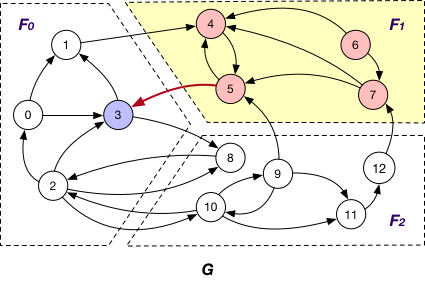OnlyIn

Under this case, each fragment Fi maintains “local” nodes v and a set of “mirrors” for nodes v’ in other fragments such that there exists an edge (v’, v). In graph G, F1 maintains “mirror” nodes {1, 9, 12} besides its local nodes.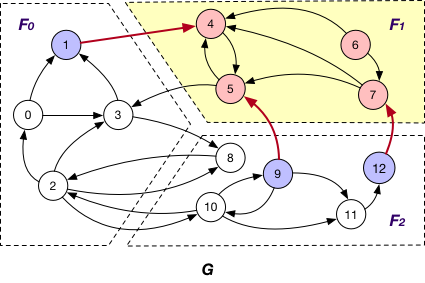BothInOut

Each fragment Fi maintains “local” nodes v and a set of “mirrors” for nodes v’ in other fragments such that there exists an edge (v, v’) or (v’, v). Hence, in graph G, “mirror” nodes {1, 3, 9, 12} are stored in F1 when BothInOut is applied.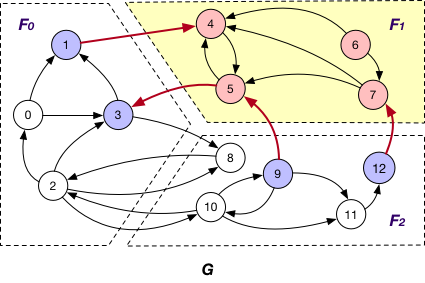PartitionStrategy

Edge Cut

An edge cut partitioning splits vertices of a graph into roughly equal size clusters. The edges are stored in the same cluster as one or both of its endpoints. Edges with endpoints distributed across different clusters are crossing edges.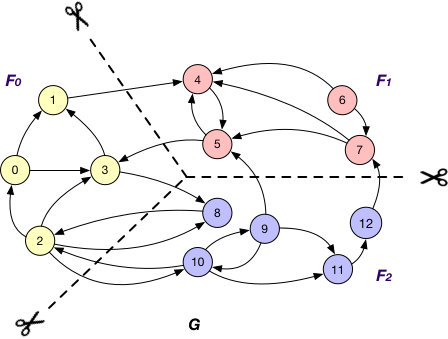Vertex Cut

A vertex-cut partitioning divides edges of a graph into roughly equal size fragments. The vertices that hold the endpoints of an edge are also placed in the same fragment as the edge itself. A vertex has to be replicated when its adjacent edges are distributed across different fragments.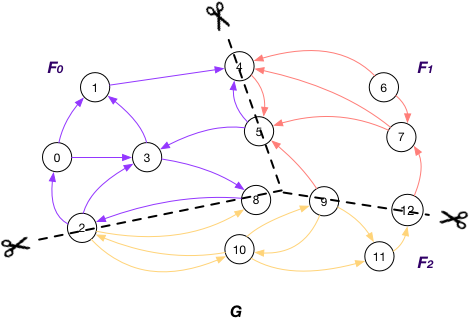Vertices on GraphScope analytical engine

A node v is referred to as an

OuterVertex

OuterVertex of fragment Fi if it resides at another fragment Fj and there exists a node v’ in Fi such that (v, v’) or (v’, v) is an edge; e.g., nodes {1, 3, 9, 12} are OuterVertex of fragment F1 in graph G;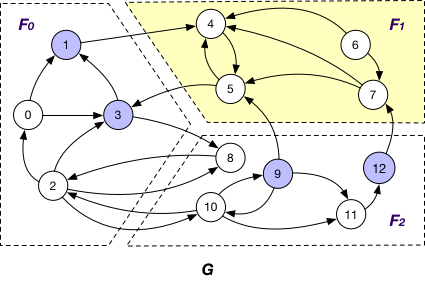InnerVertex

InnerVertex of fragment Fi if it is distributed to Fi; e.g. nodes {4, 5, 6, 7} are InnerVertex of fragment F1 in G;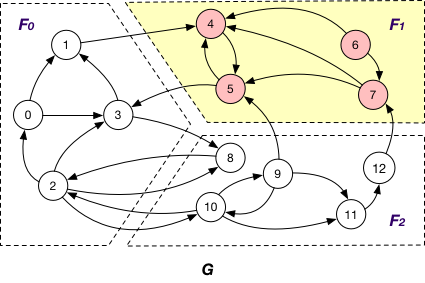InnerVertexWithOutgoingEdge

InnerVertexWithOutgoingEdge of fragment Fi if it is stored in Fi and has an adjacent edge (v, v’) outcoming to a node v’ in another fragment Fj; e.g., node 5 is an InnerVertexWithOutgoingEdge of F1 in G with the outgoing edge (5, 3);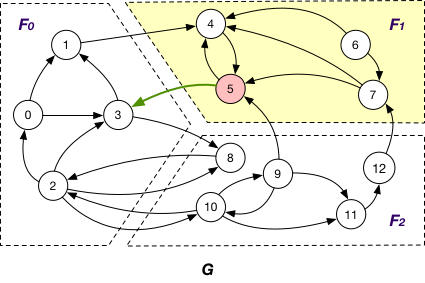InnerVertexWithIncomingEdge

InnerVertexWithIncomingEdge of fragment Fi if it is maintained in Fi has an adjacent edge (v’, v) incoming from a node v’ in another fragment Fj; e.g., nodes {4, 5, 7} are InnerVertexWithIncomingEdge of F1 in G, and (1, 4), (9, 5), and (12, 7) are corresponding incoming edges.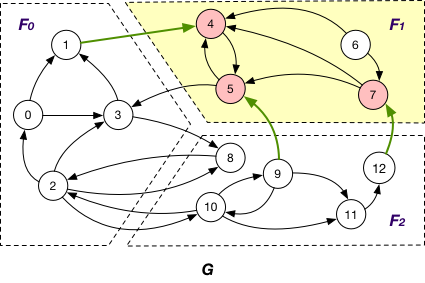MessageStrategy

Below are some message passing and synchronization strategies adopted by GraphScope analytical engine.

AlongOutgoingEdgeToOuterVertex

Here the message is passed along crossing edges from InnerVertexWithOutgoingEdge to OuterVertex. For instance, the message is passed from node 5 to 3 in graph G.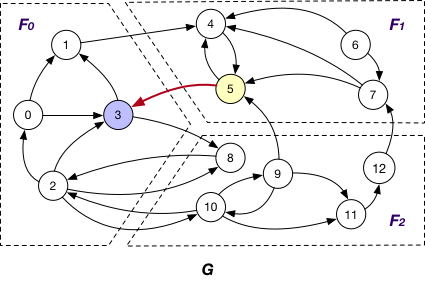AlongIncomingEdgeToOuterVertex

Under this case, the message is passed along crossing edges from InnerVertexWithIncomingEdge to OuterVertex. For example, the message is passed from node 5 to 9 in graph G.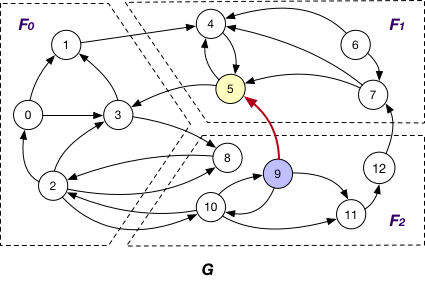AlongEdgeToOuterVertex

Each message is passed along crossing edges from nodes that are both InnerVertexWithIncomingEdge and InnerVertexWithOutgoingEdge to OuterVertex, e.g., messages are passed from node 5 to 3 and 9 and vice versa in graph G.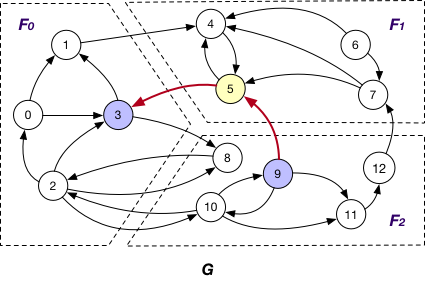SyncOnOuterVertexAsTarget

It is applied in company with the OnlyOut loading strategy. Here each fragment Fi sends the states of its “mirror” node of OuterVertex v to Fj that v resides, if there exists edge (v’, v) and v’ is “local” node of Fi, for synchronizing different states of v. For instance, the state of “mirror” node 3 is sent from F1 to F0 for synchronization at F0.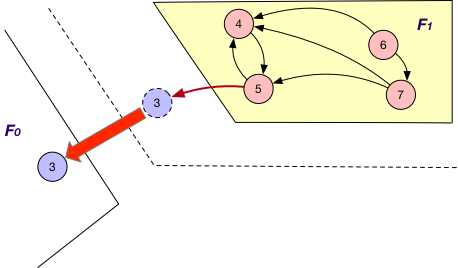SyncOnOuterVertexAsSource

It is applied together with the OnlyIn loading strategy. Similar to SyncStateOnOuterVertexAsTarget, each fragment Fi sends the states of its “mirror” nodes v to the corresponding fragments for synchronization. The difference is that for each such “mirror”, there exists outgoing edge (v, v’) to certain “local” node v’ of Fi. For example, the states of “mirror” nodes 1, 9, and 12 are sent from F1 to F0 and F2 for synchronization with other states.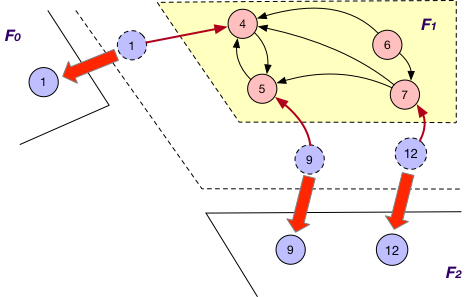SyncOnOuterVertex

This is applied together with the BothInOut loading strategy. Under this case, each fragment Fi sends the states of all its “mirror” nodes v to the corresponding fragments for synchronization, regardless of the directions of edges adjacent to v, e.g., the states of “mirror” nodes 1, 3, 9 and 12 are sent from F1 to F0 and F2 for further synchronization.